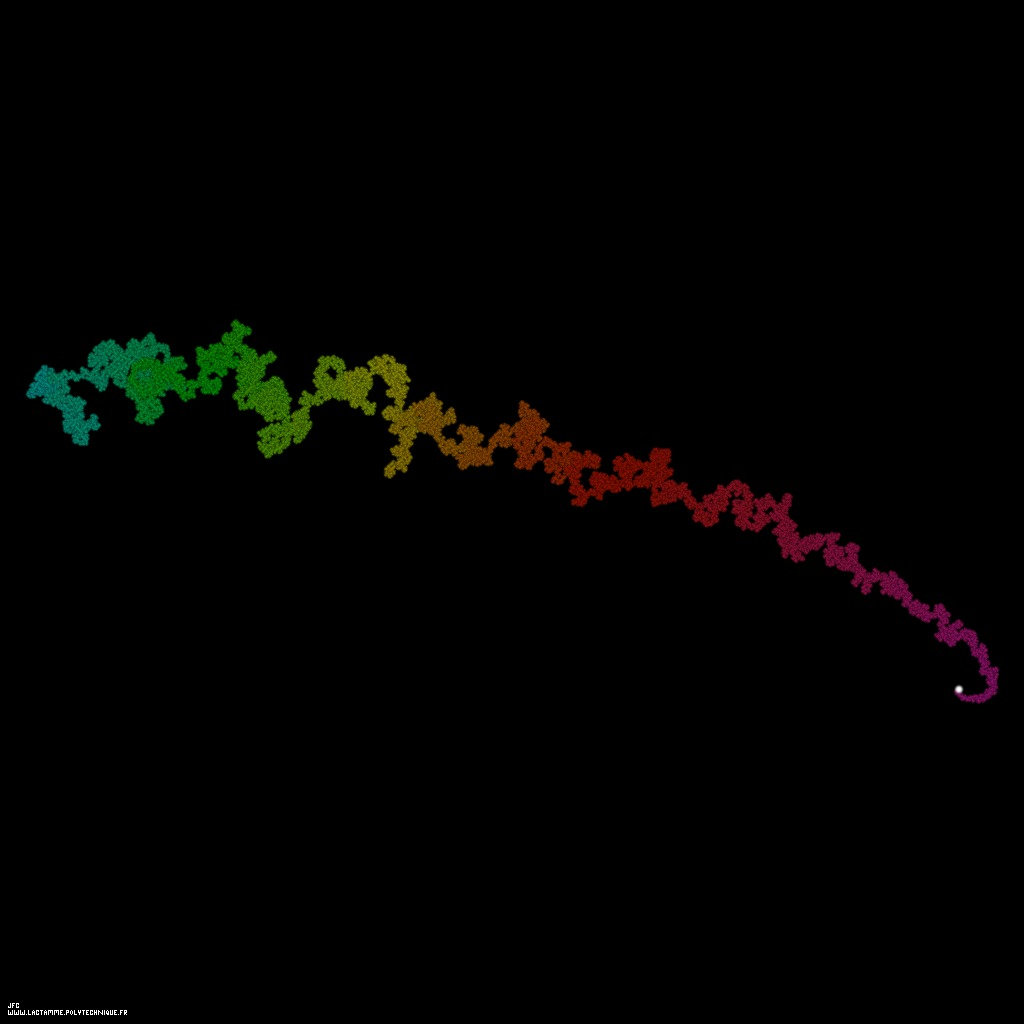The special Liouville function displayed as a bidimensional random walk for the integer numbers from 2 to 100001 [La fonction spéciale de Liouville visualisée comme une marche aléatoire bidimensionnelle pour les nombres entiers de 2 à 100001].

This picture displays the special Liouville function as a bidimensional random walk. For each integer number 'n' the function DPD(n) gives the number of different prime divisors; for example:
```                    DPD(2) = 1
DPD(3) = 1
DPD(4) = 1
DPD(5) = 1
DPD(6) = 2
DPD(7) = 1
DPD(8) = 1
DPD(9) = 1
(...)
```
Let's recall that 1 is not a prime number when 2 is the first one (and the only even one...).

The special Liouville function sL(n) equals:
```                                DPD(n)
sL(n) = (-1)
```
Hence:
```                    sL(2) = -1
sL(3) = -1
sL(4) = -1
sL(5) = -1
sL(6) = +1
sL(7) = -1
sL(8) = -1
sL(9) = -1
(...)
```

Then one defines the following dynamics:
```                    X(0) = 0
Y(0) = 0
```
```                    X(i+1) = X(i) + L(2*i+2)
Y(i+1) = Y(i) + L(2*i+3)
```
(the point {X(0),Y(0)} is on the right of the picture -white point-, when the colors used {magenta,red,yellow,green,cyan} are an increasing function of 'i')

It is useful to compare this trajectory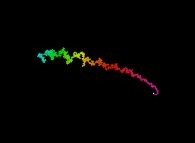with the one of the Liouville function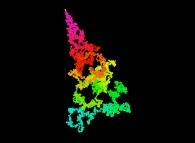.

See the superposition of three different computations: the integer numbers from 2 to 200001 (Red), 2 to 400001 (Green) and 2 to 800001 (Blue)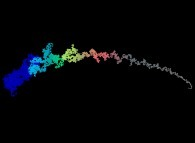and another superposition of three different computations: the integer numbers from 2 to 400001 (Red), 2 to 800001 (Green) and 2 to 1600001 (Blue)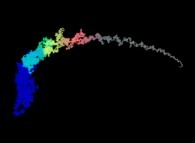.

(CMAP28 WWW site: this page was created on 01/10/2010 and last updated on 03/03/2016 07:42:30 -CET-)

[See all related pictures (including this one) [Voir toutes les images associées (incluant celle-ci)]]

[Please visit the related NumberTheory picture gallery [Visitez la galerie d'images NumberTheory associée]]
[Go back to AVirtualMachineForExploringSpaceTimeAndBeyond [Retour à AVirtualMachineForExploringSpaceTimeAndBeyond]]
[The Y2K bug [Le bug de l'an 2000]]

[Site Map, Help and Search [Plan du Site, Aide et Recherche]]
[Mail [Courrier]]
[About Pictures and Animations [A Propos des Images et des Animations]]